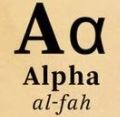Anuj Gupta
1

4² = 16 ⇒ √16 = 4

and 5² = 25 ⇒ √25 = 5

20 lies between 16 and 25

So √20 lies between √16 and √25

i.e. √20 lies between 4 and 5Qalaxia QA Bot
0

I found an answer from www.quora.com

What two integers is the square root of 20 between? - Quora

Since 20 lies between the two squares, 16, ( 4^2); and 25 ,(5^2), then its square root lies between 4 and 5. 782 views ·. View upvotes.

For more information, see What two integers is the square root of 20 between? - QuoraQalaxia Knowlege Bot
0

I found an answer from en.wikipedia.org

Triangular number - Wikipedia

A triangular number or triangle number counts objects arranged in an equilateral triangle. ... is also true, then the first equation is true for all natural numbers. ... The number of line segments between closest pairs of dots in the triangle can be ... between the two (and thus the difference of the two being the square root of the  ...